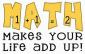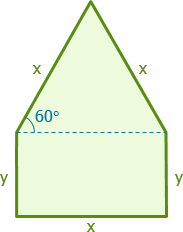# help: window consist of a rectangle with an equilateral triangle resting on its toppls help me to solve this problems as well as the solution:

1. a large window consist of a rectangle with an equilateral triangle resting on its top. if the perimeter P of the window is fixed at 33 ft, find the dimension of the triangle that will maximize the area of the window.

2. A student club on a college charges monthly membership dues of P10, less 5 centavos for each member over 60. How many members would give the club, the most revenue from the monthly dues?### #1

Solution to #1
$3x + 2y = 33$

$y = \frac{33}{2} - \frac{3}{2}x$$A = xy + \frac{1}{2}x^2 \sin 60^\circ$

$A = x \left( \frac{33}{2} - \frac{3}{2}x \right) + \frac{1}{4}\sqrt{3}x^2$

$A = \frac{33}{2}x - \frac{3}{2}x^2 + \frac{1}{4}\sqrt{3}x^2$

$A = \frac{33}{2}x - \left( \frac{3}{2} - \frac{1}{4}\sqrt{3} \right) x^2$

$\dfrac{dA}{dx} = \frac{33}{2} - 2\left( \frac{3}{2} - \frac{1}{4}\sqrt{3} \right) x = 0$

$\frac{33}{2} = 2\left( \frac{3}{2} - \frac{1}{4}\sqrt{3} \right) x$

$x = \dfrac{\frac{33}{2}}{2\left( \frac{3}{2} - \frac{1}{4}\sqrt{3} \right)}$

$x = 6 + \sqrt{3} = 7.732 ~ \text{ft}$### thank you sir for the help.

thank you sir for the help. kanang #2 sir pwede ko mangayu ug idea on how to solve?
thank you again sir....

## Add new comment

### Deafult Input

• Allowed HTML tags: <img> <em> <strong> <cite> <code> <ul> <ol> <li> <dl> <dt> <dd> <sub> <sup> <blockquote> <ins> <del> <div>
• Web page addresses and e-mail addresses turn into links automatically.
• Lines and paragraphs break automatically.
• Mathematics inside the configured delimiters is rendered by MathJax. The default math delimiters are $$...$$ and $...$ for displayed mathematics, and $...$ and $...$ for in-line mathematics.

### Plain text

• No HTML tags allowed.
• Lines and paragraphs break automatically.